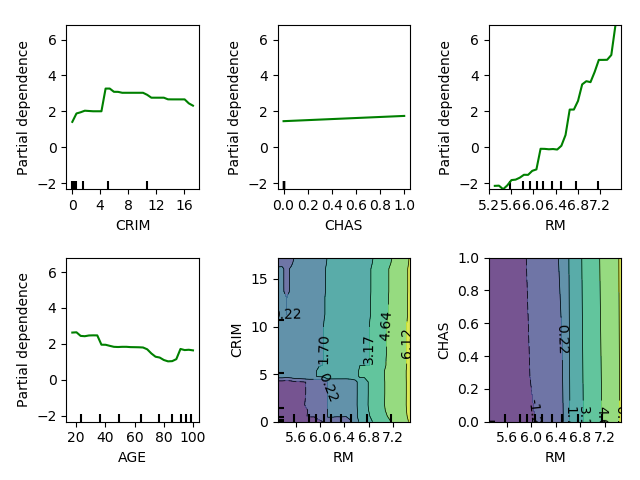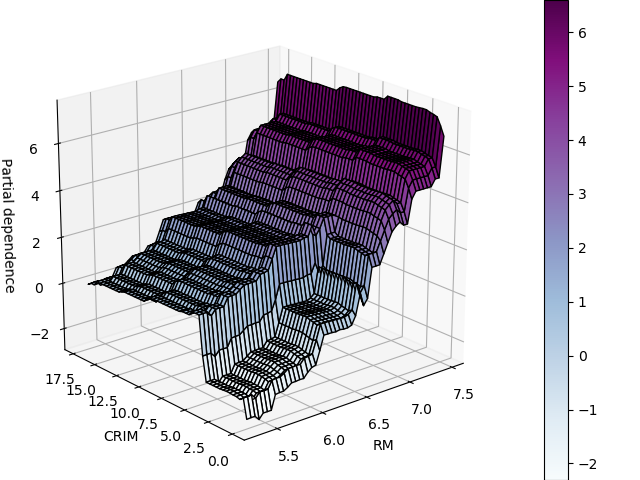# 2.3. Partial dependency plots¶

Partial dependency plots consists in evaluating the model prediction while varying one of more features and marginalizing (averaging) out the effect of the other features.

They can be computed by varying only one feature, or more

```# Load and prepare the data
from sklearn import datasets

from sklearn.model_selection import train_test_split
X_train, X_test, y_train, y_test = train_test_split(boston.data,
boston.target,
test_size=0.2,
random_state=1)
```

Train a GBRT

```from sklearn.ensemble import GradientBoostingRegressor
learning_rate=0.1, loss='huber',
random_state=1)
clf.fit(X_train, y_train)
```

## 2.3.1. All-in-one plotting function¶

```from sklearn.ensemble.partial_dependence import plot_partial_dependence
import matplotlib.pyplot as plt
```

Inspect feature 0, 3, 5, 6, and the interaction between 5 and 0, and 5 and 3

```target_features = [0, 3, 5, 6, (5, 0), (5, 3)]
fig, axs = plot_partial_dependence(clf, X_train, target_features,
feature_names=boston.feature_names,
grid_resolution=30)
plt.tight_layout()
```## 2.3.2. Lower-level partial_dependence function¶

```target_feature = (5, 0)
from sklearn.ensemble.partial_dependence import partial_dependence
partial_deps, grid = partial_dependence(clf, target_feature,
X=X_train, grid_resolution=50)
import numpy as np
# The 2D coordinate grid (for plotting)
XX, YY = np.meshgrid(grid, grid)

# Reshape the partial deps on the grid
Z = partial_deps.reshape(list(map(np.size, grid))).T
```

3D plotting

```from mpl_toolkits.mplot3d import Axes3D

fig = plt.figure()
ax = Axes3D(fig)
surf = ax.plot_surface(XX, YY, Z, rstride=1, cstride=1,
cmap=plt.cm.BuPu, edgecolor='k')
ax.set_xlabel(boston.feature_names[target_feature])
ax.set_ylabel(boston.feature_names[target_feature])
ax.set_zlabel('Partial dependence')
#  pretty init view
ax.view_init(elev=22, azim=230)
plt.colorbar(surf)
```Total running time of the script: ( 0 minutes 0.863 seconds)

Gallery generated by Sphinx-Gallery